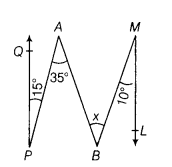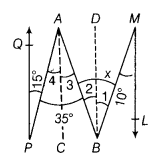# In the given figure, QP\\ML and other angles are shown. Find the value of x

In the given figure, QP\ML and other angles are shown. Find the value of x.Draw AC || QP and BD || LM.∴QP || LM=>AC || BD
and AC || QP => ∠4 = 15° …(i)
[alternate interior angles]
Also, BD|| ML=> ∠1 = 10°
[alternate interior angles]
and AC || BD => ∠2 = ∠3
[alternate interior angles]
Now, as ∠3 + ∠4 = 35°
∴∠3 + 15° = 35° [from Eq. (i)]
=> ∠3 = 20°
=> ∠2 = 20° [∵∠2 = ∠3]
Now, x = ∠1+ ∠2 = 10° +20° = 30°
Hence, the value of x is 30°.